# How to Use Scientific Calculator

Regardless of whether you’re looking to do plain arithmetic or want to solve complex science and engineering problems, you can rely on a scientific calculator to help.

Getting acquainted with a scientific calculator’s functions can be challenging. Going through the manual is an option, but the instructions can sometimes seem needlessly convoluted.

Here’s an easy guide that will teach you how to use a scientific calculator in minutes.

# How to Use a Scientific Calculator

Understanding what a scientific calculator is and its components will help you get the hang of using it quicker.

## What is a Scientific Calculator?

A scientific calculator is a digital calculator loaded with several functions, enabling the user to solve complex science, engineering, and mathematics problems.

Basic and business calculators only allow you to do basic operations like addition, subtraction, multiplication, and division. In contrast, scientific calculators enable you to quickly carry out trigonometric functions, work with natural logarithms and square roots, and plot graphs.

Furthermore, the calculators also allow you to work with scientific notation and exponents. Unlike simple calculators, the order of operations rule is applied to all calculations you do on scientific calculators.

While these calculators come with a lot of added functionality, they are also priced considerably more than basic and business calculators.

### Easiest Scientific Calculator to Use

You don’t always need to buy and use a scientific calculator to solve problems. Meta-Calculator.com is a web-based, free-to-use scientific calculator that comes loaded with all the features available in expensive graphing calculators.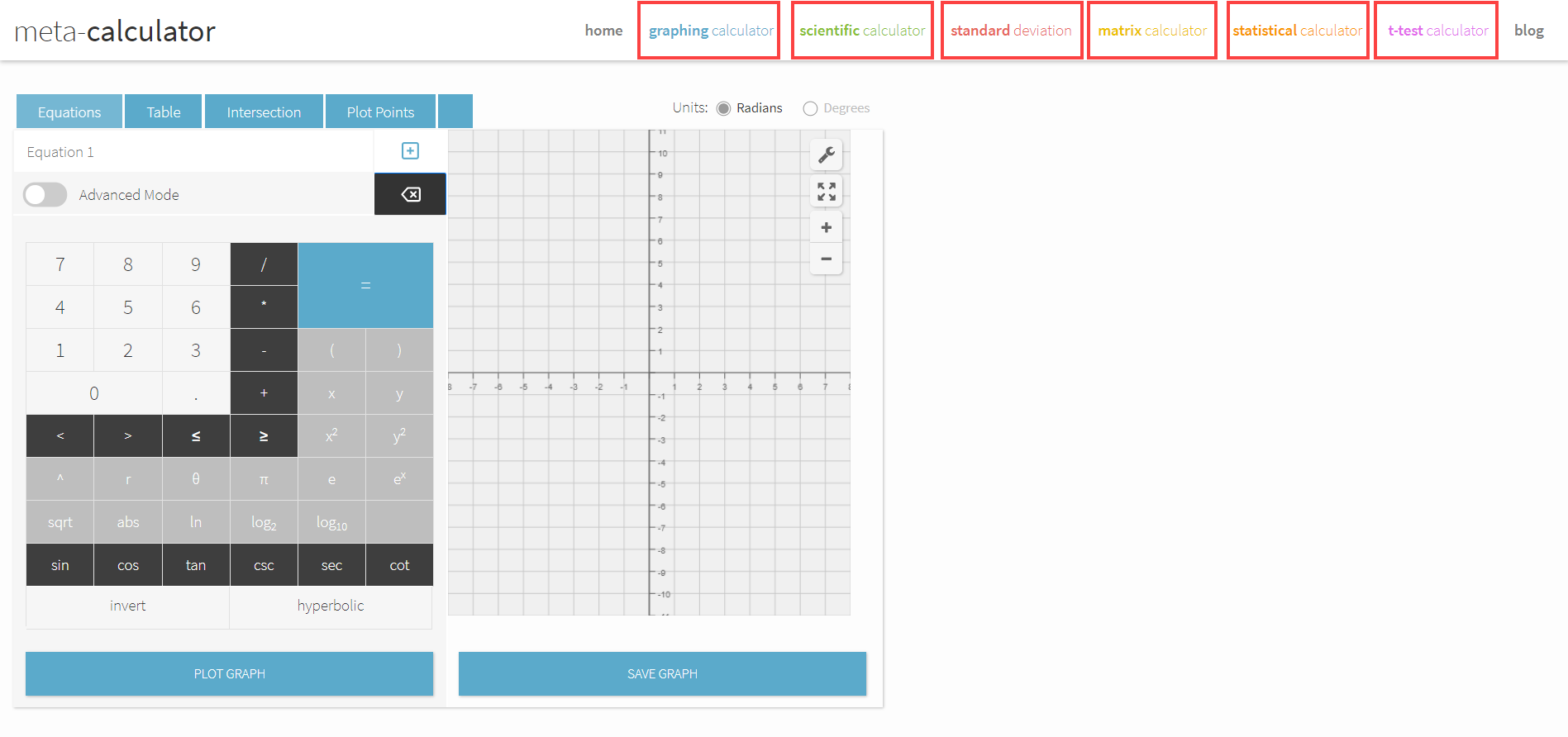Besides enabling you to find standard deviations of data, it also allows you to do matrix calculations, carry out t-tests, and plot coordinates on graphs. You can use it on your phone, tablet, and computer.

Furthermore, you don’t need to use complex key sequences to access features like you would in a traditional scientific calculator. You can use the calculator to solve complex science and math problems with just your mouse.

### What A Scientific Calculator Looks Like

The position of the keys on scientific calculators varies by make and model. That being said, most scientific calculators have a similar design and feature identical functions.

A typical scientific calculator looks like this: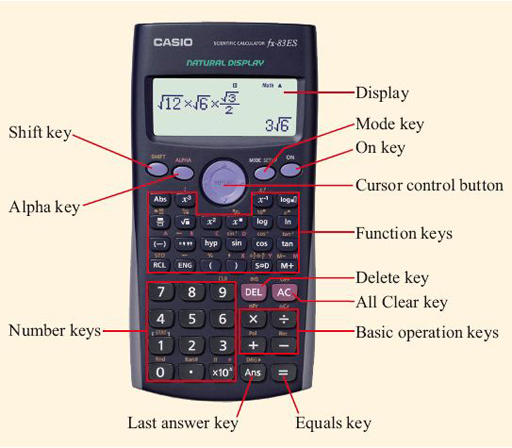Casio FX-991EX

To use a scientific calculator, you must first switch it on by pressing the “ON” button, usually on the corner of the top row of keys on the calculator. When the calculator is turned on, a flashing cursor will appear on the calculator display.

You can then proceed to apply any of the several operations that your scientific calculator comes loaded with.

### The Keys on A Scientific Calculator

The lower half of the typical scientific calculator features the number keys and the keys required to perform basic arithmetic. Besides the number keys, you will find the + – x and / keys.

You will also find the = key on the bottom row. It is the key you need to press to display the result of the expression you’ve entered.

We’re using the Casio FX-991EX for the purpose of this tutorial. Othe parenthesis keys are in the row above the number keys. Using parenthesis can help you feed in the exact expression you want to be worked out.

You must note that most keys on scientific calculators have more than one function. The primary function is stamped on the keys; the second function of the key is printed above the key.

You can use the function printed above any by pressing the SHIFT key before pressing it.

Some keys also have a third function, printed above the key in red font. These functions allow you to store calculated values in the device’s built-in memory. The values can be retrieved whenever required. We cover using the calculator’s memory in detail later in the post.

While keys provide access to most of the calculator’s features, some options can only be accessed via menus. For instance, the MODE menu may allow you to pick from several options: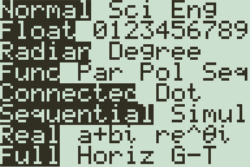## Important Scientific Calculator Functions

In the following sections, we’ve highlighted all of the important scientific calculator functions and broken down how to use them.

### Basic Calculations

To do basic calculations with your scientific calculator, you must enter the expressions in the exact order as you would write it on paper.

After entering your expression, pressing the = key will display the answer on the screen.

You can enter an expression that is too long for the screen to display. In this case, you can use the arrow keys to scroll left and right on the calculator.

Typing a long expression into the calculator will sometimes cause the shape of a cursor to change to a block. If you see the cursor change to a block, it means that your calculator only allows entering that many characters at a time. You will need to break up your expression for the calculator to solve it.

You can also enter negative numbers into the calculator by simply pressing the “-” key on the keyboard.

### Making Corrections

Getting the hang of the position of the keys takes time, and making mistakes is common. You don’t have to type in the entire expression again if you make a mistake.

If you accidentally enter a wrong digit or symbol, you can use the arrow keys and the “DEL” key to remove the digit or symbol.

However, in some scenarios, it makes more sense to clear the entire expression. If you find yourself wanting to start over with feeding the expression into the calculator, press the “AC” button.

Sometimes, pressing the wrong key when entering an expression into the calculator can lead to a syntax error. Some calculators give you the option to “Quit” or “Clear Screen” directly, like so:In other calculators, you will need to start over with entering the expression by pressing “AC” or using the arrow keys to fix the erroneous expression.

### Working with Exponents

There are many ways of working with exponents on a scientific calculator. Most calculators come with x2 and x3 that you can use to work with powers. For instance, if you wanted to square three, you would press the “3” key and press the x2 button after.

You can also work with bigger exponents on a scientific calculator using the x button. To use it, first, enter the base number (let’s say it’s 2) and then press the x key.

A box will appear above the base number, which you can navigate to using the arrow keys. While navigating, when you see the exponent box with a flashing cursor (“|”), you can enter the exponent number in it.

Use your arrow keys to move your cursor away from the exponent box, enter more numbers, and add more operations to the calculation.

It is important to note that some scientific calculators have a “^” key instead of the x key. The two have the same functionality, so you can use them in the same way. Some calculators do not have x2 and x3 keys, so you will need to use the ^ or x key to work with exponents.

### Working with Fractions

Working with fractions on a scientific calculator is as simple as pressing the “/” button. After entering the symbol, you can use the arrow keys to navigate the numerator and denominator positions and enter expressions.

The fraction template that appears will look like this: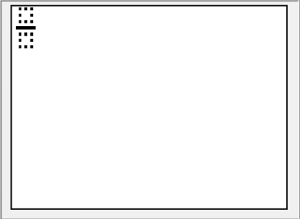After you fill in the numerator, pressing the down arrow key will move the cursor to the bottom of the template. You can enter the denominator here. You could then either press the = key to calculate the result. You could also hit the right arrow key, move out of the denominator, and input the rest of the calculation.

Some calculators do not have the “/” button, but it is possible to work with fractions on these calculators as well. You will need to use the parenthesis buttons to separate your fractions and get a result. For instance, to calculate 3/11 + 4/13 , you will need to enter (3/11) + (4/13) in the calculator.

### Working with Scientific Notations

Using scientific notation enables you to work with numbers too large or too small to represent regularly. Every scientific calculator will automatically display a result in scientific notation if the result is greater than or equal to 1010.

The same logic applies to small numbers. If a result is too small, it will be represented in scientific notation. The calculator’s mode decides how small the number needs to be for being represented in scientific notation. You can find details about this in your calculator’s manual.

To enter scientific notations on your calculator, you can use the x 10x key. For instance, if you want to enter 2.37 x 107 in your calculator, you must first enter 2.37, press the x 10x key, and then set the exponent to 7.

### Finding Roots on Your Calculator

Just like there are keys on the calculator to enter exponents, there are also keys that enter roots. To calculate the square root of a number, press the “√” button, and then enter the number you want to calculate the root of.

Some calculators enable you to calculate the cube root of a number using the alternate function of the “√” button. If your calculator does not provide this alternate function, you can calculate higher roots using the “√∎” button on the calculator.

Pressing the button will make a template appear, and you must use the arrow keys in the same way you would with the fraction template and enter the desired numbers.

### Finding Trigonometric Ratios

You can measure an angle in various units. You can work with degrees, radians, and grads on your scientific calculator.

If you want to calculate the trigonometric ratios in degrees, make sure that the calculator is set to use those units. You can determine what unit your calculator is set to by looking at the top portion of the display. For example, if you see a “D,” it’s set to degrees.

On the other hand, if you see an “R” or a “G,” the calculator is set to provide you results in radians and grads, respectively. To switch to the unit you prefer, you will need to access the MODE menu by pressing the “MODE” button.

You can use the arrow keys to highlight the unit you want to use and then hit the = button to exit.

To apply the cos, tan, or sine function, you must press the cos, tan, or sin button, respectively. The symbol will appear on the screen.

Next, you must feed the size of the angle into the calculator. You don’t need to close the parenthesis unless you are using the ratio as a part of a larger calculation. Pressing the = key will calculate the angle and display the results.

You can also determine inverse trigonometric values on your calculator by using the alternate functions of the sin, cos, and tan keys. The operations can be applied similarly to how standard trigonometric calculations are done.

### Applying Logarithms

Calculating a logarithm to the base ten is as simple as pressing the log key and then entering the number that you want to calculate the log of. Pressing the = key will display the result.

You must note that, like with trigonometric functions, using the logarithm function will also open up a bracket. The bracket must be closed if you want to enter a longer calculation.

Scientific calculators make working with natural logarithms easy too. The “ln” key works just like the “log” key. All you have to do is press the button and then enter the number that you want to calculate the logarithm of. You will need to close the bracket that appears to enter an extended expression.

You must bear in mind that some calculators do not have an “ln” key and have a “log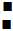key. To calculate the natural logarithm with this key, you must enter the Euler’s constant in the base of the template.

### Storing Values in Calculator Memory

The memory built into a scientific calculator is one of its most useful features. Solutions to science and math problems can often be lengthy, and the ability to store values after calculating them make arriving at the final result easy.

Most scientific calculators have several memories. The “M” memory allows you to store one value in it, and the memory can be accessed by using the “M+” key.

#### Clearing Stored Values

Clearing any values stored in memory previously is a good idea before using the memory. The Casio FX-991EX requires you to press the shift button, followed by the nine key to access the “CLR” function. You must then press the “2” key to indicate memory and confirm with the = button. You can then return to the home screen pressing AC.

Using this key sequence will clear values in all of the memories of the calculator. If you’re using a different calculator, the key sequence to clear memory may be different. Going through your calculator’s manual is the best way to learn the right way of clearing the calculator’s memory.

#### Storing New Values

To store a result you’ve calculated in the “M” memory, press the SHIFT button, then RCL, and then M+. We are using the alternate function of the RCL key (called ‘STO,’ short for “store”).

After activating the store option, you must indicate to the calculator where the value must be stored. To store it in memory “M,” press the M+ button.

You can perform several operations with the value stored in memory “M.” To add the stored value to any expression, press the M+ button. To subtract the M value, press the SHIFT button and then the M+ button.

Inserting the letter “M” into any expression is as simple as pressing ALPHA and then the M+ button. This way, you can perform operations on the results you’ve already calculated with ease.

For example, you can square the value stored in the memory “M” by using the entering pressing ALPHA, then M+, and then hitting the x2 and = keys to complete the operation.

You can store values in the six other memories in the calculator, too. After activating the store function, all you need to do is press the ALPHA button and pick from the six alphabets you want to store the data in.

## Conclusion

Now that you’ve gone through this guide, trying to apply some of the operations yourself is the best way to get the hang of using a scientific calculator.

After some practice, you will understand what buttons you need to press to perform which operations. You will also get a feel for the order in which you have to press keys to use different functions.

In only a few minutes of practice, you will be able to use the calculator to solve complex problems quickly.

We have various comprehensive calculators that you can use online for free. You can choose from t-test calculator, graphing, matrix, the standard deviation to statistics, and scientific calculators. Check it here